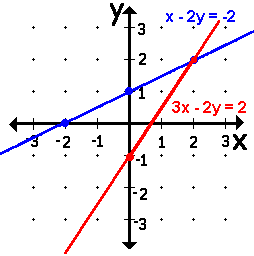### Solving Systems of Equations   © 2004, A2

A system is "a set of equations or statement which are to be solved simultaneously" (at the same time). That means the solution, answer, to the system is the solution to EVERY equation in the system.

For a system of linear equations, there are three kinds of answers: 1 solution, no solution, or many solutions. The conditions which lead to each kind of answer are examined below.

There are many methods of solving the system. This site includes pages on solving linear systems by

Solve A System Graphically

To solve a system graphically, find the point of interesection of the lines.

Because it is not always easy to determine where the graphs intersect, this method may not always be used, so, another system must be used to find the point of interesection of the lines, the solution.

 sample graphsystem: x-2y=-23x-2y=2 x-2y=-22x-2y=2 x-2y=-24x-8y=-8 described as: independent inconsistent dependent lines are: intersecting parallel coincident solution: (2,2), the point of interesection x=8/0 and y=4/0 are undefined, because division by 0 is undefined.The is no solution.The lines don't intersect. SOME of the solutions are:(-2,0), (0,1), (2,2).

Solve A System Mentally

A system may be solved mentally, only if one can recognize parallel or coincident lines.

If one line is a multiple of the other, the lines are coincident and have all points in common. An ordered pair for one point is an ordered pair for the other line and a solution to the system. The x-intercept, (?, 0), or the y-intercept, (0,?) are good points to obtain to solve the system.

If lines are parallel, they do not intersect so the system has no solution. Recognizing that lines are parallel even before they are graphed is very time efficient.

If a line is written y= mx + b, the y-intercept is b and the slope is m.

If a line is written as Ax + By = C, the slope of the line is equal to -A/B.

If a line is written as Ax + By = C, the y-intercept is equal to C/B.

If each line in the system has the same slope but a different y-intercept, the lines are parallel and there is no solution.

If each line in the system has the same slope and the same y-intercept, the lines are coincident.

 Solve the system. Mouseover the arrow to check answer. x - 2y = -2-3x - 6y = -6y = -2x + 4y = -2x + 55x + 5y = 1010x + 10y = 12y = 4x - 18y = 8x + 8© 2/18/2011 mathnstuff.com/math/algebra/amental.htm